## Vba nested loops in Excel

Nested loop is nothing but a loop within a loop. It is a double loop. You can make a triple loop and q quadruple loop.

There may be any number of loops within a loop, but the loops has to be properly nested without any conflict.

This feature of Excel is very useful in comparing a range of cells and is most often used in Excel Programming.

The following example illustrates the nested loop.

The code compares each value of a cell in column A with that of column B.

1. Sub Nested_Loop()
2. Dim i, j As Integer
3. For i = 1 To 2
4. For j = 1 To 2
5. If Sheets(1).Range("A" & CStr(i)) = Sheets(1).Range("B" & CStr(j)) Then
6. MsgBox "A" & CStr(i) & " and B" & CStr(j) & " are Same"
7. Else
8. MsgBox "A" & CStr(i) & " and B" & CStr(j) & " are different"
9. End If
10. Next j
11. Next i
12. End Sub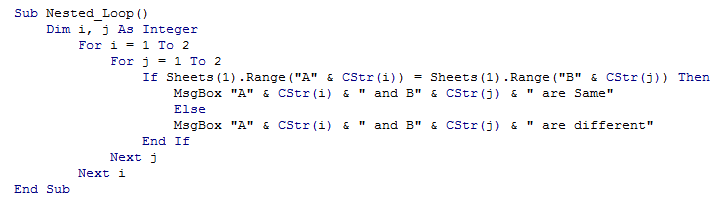The above code compares A1 to A2 with that of B1 to B2 and the result is as shown below

A1 and B1 are different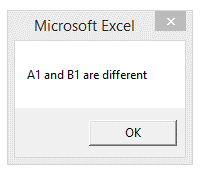A1 and B2 are different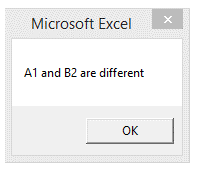A2 and B1 are different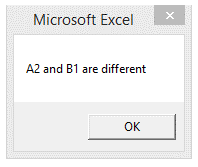A2 and B2 are different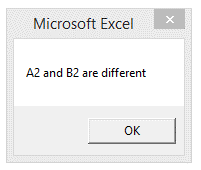Note the placement of "Next" statement in the example. The latest loop should be closed first